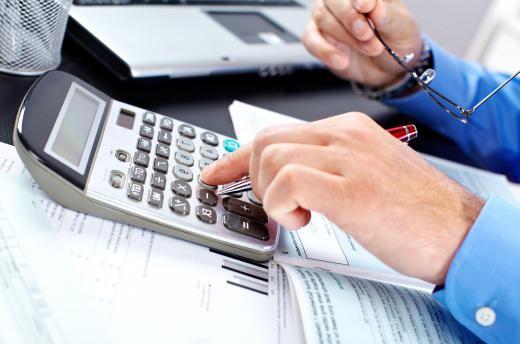Finance
Fact Checked

# What is Diminishing Balance Depreciation?

Osmand Vitez
Osmand Vitez

Diminishing balance depreciation is an accounting tool that allows companies to reduce the book value of fixed assets. Fixed assets are considered long-term assets meant to help companies accomplish goals for earning profit when selling goods and services to consumers. Fixed assets like equipment and machinery, while long-term, must eventually be replaced, and thus depreciate over the useful life of the item. The purpose of the diminishing balance depreciation method is to apply a set percentage of depreciation each year so the company can accurately calculate the asset’s value at a particular time.

To calculate the depreciation of a fixed asset, companies must first determine the salvage value of the item, or the amount a company could sell an item for after it's estimated useful life. For example, a machine that produces widgets may have a 10 year useful life. At the end of this period, the company can sell the asset for an amount based on the price of same or similar machinery available for sale that also is 10 years old.Diminishing balance depreciation enables companies to calculate an asset's value at a particular time.

The percentage of annual depreciation in the diminishing balance depreciation method is based on an estimate made by a company’s management and accounting team. While different methods will exist for this calculation, a basic method is 100 percent divided by the years of useful life. The theory behind this method is that the machine is at 100 percent capacity prior to installation into the company’s operations. Once used, the asset will most likely depreciate so long as the use is consistent. For example, a machine with 10 years of useful life would depreciate 10 percent each year.

Accountants can usually figure the diminishing balance depreciation fairly easily once the basic pieces are in place. For example, if a machine costs \$150,000 USD, has a 20 year useful life, and a salvage value of zero, accountants would begin by determining the annual percentage of depreciation at five percent (100 / 20). To figure the annual dollar depreciation amount for the machine, accountants will multiply the asset’s book value times the annual depreciation percentage. Therefore, the annual depreciation for year one on the machine is \$7,500 USD, with the machine's adjusted book values at \$142,500 USD. For year two, the annual dollar depreciation is \$7,125 (142,500 x .05). This continues until the asset reaches a zero salvage value on the company’s accounting books.

The result of the diminishing balance depreciation is quite evident. The annual depreciation figure slowly reduces each year, reflecting the lower asset value from previous years’ depreciation adjustments. In theory, accountants believe it represent a more accurate picture of the asset’s depreciable value.

## You might also Like